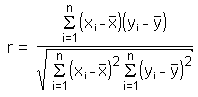Definition

Pearson's Correlation Coefficient measures the strength of the linear relationship between two variables. It is usually signified by r and takes on values between -1.0 and 1.0. Where -1.0 is a perfect negative correlation, 0.0 is no correlation, and 1.0 is a perfect positive correlation.

The coefficient also tells you if the relationship is positive or negative by whether the value of r is positive or negative. Positive correlation indicates that as the values of x increase, the values of y also increase. A negative correlation indicates that as one variable increases, the other decreases.

The formula for r-value is as follows:Mostly calculators and computers are used to find the coefficient. It is the expectation of this course that you will understand how to use and interpret the coefficient, not calculate it using the formula.

Listen